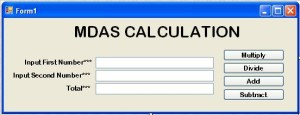0
3598

# How to Create the MDAS Calculator in VB.Net

In this 2019 tutorial about VB.Net Code For Calculator will teach you a step by step process on how to create an MDAS Calculator in VB.NET Code with examples.

MDAS is the four fundamentals operation in solving the mathematical equation. MDAS stands for MULTIPLICATION, DIVISION, ADDITION, and SUBTRACTION.
I’m going to use Visual Studio 2008 for creating this desktop application.

The VB.Net Code For Calculator use in this tutorial can run in any higher version of VS

## Let’s begin the VB.Net Code For Calculator :

Open the Visual Studio 2008 and create a new Windows Form Application. After that, set the Form just like this.After setting up the Form, double click the “Multiply” Button and set up the formula for the multiplication method.

And then, these are the formula for the Divide, Add and Subtract Buttons.

After setting up the formula in each corresponding Buttons, go back to the Form Design, double click the Form and disable the TextBox(txttot) in the first load of the Form.

Finally, these are the full codes that you have made.